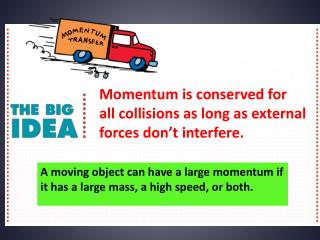Download PresentationMomentum is conserved for all collisions as long as external forces don’t interfere.

# Momentum is conserved for all collisions as long as external forces don’t interfere. - PowerPoint PPT PresentationDownload Presentation## Momentum is conserved for all collisions as long as external forces don’t interfere.

- - - - - - - - - - - - - - - - - - - - - - - - - - - E N D - - - - - - - - - - - - - - - - - - - - - - - - - - -
##### Presentation Transcript

1. Momentum is conserved for all collisions as long as external forces don’t interfere. A moving object can have a large momentum if it has a large mass, a high speed, or both.

2. Momentum • It is harder to stop a large truck than a small car when both are moving at the same speed. • The truck has more momentum than the car. By momentum, we mean inertia in motion. • A moving truck has more momentum than a car moving at the same speed because the truck has more mass. • A fast car can have more momentum than a slow truck. • A truck at rest has no momentum at all.

3. Momentum A truck rolling down a hill has more momentum than a roller skate with the same speed. But if the truck is at rest and the roller skate moves, then the skate has more momentum.

4. Momentum think! Can you think of a case where a roller skate and a truck would have the same momentum? Answer:The roller skate and truck can have the same momentum if the speed of the roller skate is much greater than the speed of the truck. For example, a 1000-kg truck backing out of a driveway at 0.01 m/s has the same momentum as a 1-kg skate going 10 m/s. Both have momentum = 10 kg•m/s.

5. What factors affect an object’s momentum? Momentum is the mass of an object multiplied by its velocity. momentum = mass × velocity momentum = mv p=mv When direction is not an important factor, momentum = mass × speed

6. Assessment Question • When the speed of an object is doubled, its momentum • remains unchanged in accord with the conservation of momentum. • doubles. • quadruples. • decreases. Answer: B

7. 8.4Conservation of Momentum The law of conservation of momentum states that, in the absence of an external force, the momentum of a system remains unchanged.

8. Conservation of Momentum The momentum before firing is zero. After firing, the net momentum is still zero because the momentum of the cannon is equal and opposite to the momentum of the cannonball. • The force on the cannonball inside the cannon barrel is equal and opposite to the force causing the cannon to recoil. The action and reaction forces are internal to the system so they don’t change the momentum of the cannon-cannonball system. • Before the firing, the momentum is zero. • After the firing, the net momentum is still zero. • Net momentum is neither gained nor lost.

9. Conservation of Momentum think! Newton’s second law states that if no net force is exerted on a system, no acceleration occurs. Does it follow that no change in momentum occurs? Answer: Yes, because no acceleration means that no change occurs in velocity or in momentum (mass × velocity). Another line of reasoning is simply that no net force means there is no net impulse and thus no change in momentum.

10. Conservation of Momentum What does the law of conservation of momentum state?

11. Assessment Questions • The momentum relationship is a direct result of Newton’s • first law. • second law. • third law. • law of gravity. • Answer: B

12. Assessment Questions • 3. A falling firecracker bursts into two pieces. Compared with the momentum of the firecracker when it bursts, the two pieces • combined have the same momentum. • each have half as much momentum. • have more momentum. • may or may not have more momentum. Answer: A